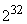Time: 3 Hours                                                                                                     Max. Marks: 100

NOTE: There are 9 Questions in all.

·      Question 1 is compulsory and carries 20 marks. Answer to Q. 1. must be written in the space provided for it in the answer book supplied and nowhere else.

·      Out of the remaining EIGHT Questions answer any FIVE Questions. Each question carries 16 marks.

·      Any required data not explicitly given, may be suitably assumed and stated.

Q.1       Choose the correct or best alternative in the following:                                         (2x10)

a.       Which of the following can be the beginning address of a block that contains 1024 addresses?

(A)  205.16.37.32                               (B)  190.16.42.0

(C)    17.17.32.0                                  (D)  123.45.24.52

b.      In ________ routing, the full IP address of a destination is given in the routing table.

(A)    next-hop                                      (B)  network-specific

(C)  host-specific                                 (D)  default

c.   The connection oriented protocol at the transport layer is

(A)     SMTP.                                        (B)  TCP.

(C)  UDP.                                           (D)  FTP.

d.   The option field of the TCP header ranges from 0 to _______ bytes.

(A)    10                                               (B) 20

(C)  40                                               (D)e.   Karn’s algorithm is used in calculations by the ______ timer.

(B)     retransmission                              (B)  persistence

(C)  keep alive                                     (D)  time-waited

f.    Synchronous transmission does not have __________

(A)     a start bit                                      (B)  a stop bit

(C)  gaps between bytes                      (D)  all the above

g.   The baud-rate for a 72,000 bps 64-QAM signal is ____________

(A)     10,000 bauds.                              (B)  11,000 bauds.

(C)  12,000 bauds.                              (D)  1200 bauds.

h.   Which multiplexing technique transmits digital signals?

(A)    FDM                                           (B)  TDM

(C)  WDM                                          (D)  None of the above

i.    Trunks are transmission media such as _________ that handle the telephone communication between offices.

(A)   Twisted-pair cable                        (B) Fiber-optic cable

(C) Satellite links                                 (D) Both (B) and (C)

j.    Which error detection method can detect a burst error?

(A)  The parity-check                          (B)  Two-dimensional parity check

(C)  CRC                                            (D)  Both (B) and (C)

Answer any FIVE Questions out of EIGHT Questions.

Each question carries 16 marks.

Q.2     a.   Match the following to one of the seven OSI layer:

(i)                  Establishes, manages and terminates the interaction between communicating systems.

(ii)                Synchronization of bits.

(iii)               Provides independence from differences in data representation.

(iv)              Responsibility for information between adjacent nodes.

(v)                Delivery from a specific process on one computer to a specific process on the other.                                   (5)

b.   Which of the following is a confirmed service, an unconfirmed service, both, or neither,

(i)   connection establishment.

(ii)  data transmission.

(iii) connection release.                                                                                       (3)

c.   What are the two reasons for using layered protocols?                                        (4)

d.   Compare the OSI reference model with TCP/IP reference model by listing two common features and two differences.                                          (4)

Q.3     a.   A signal has two data levels with a pulse duration of 1 msec.  Calculate the pulse rate and bit rate.                                                              (2)

b.   We want to digitize the human voice.  What is the bit rate, assuming 8 bits per-sample?                   (2)

c.   Given a bandwidth of 10,000 Hz (1000 to 11,000 Hz), draw the full-duplex ASK diagram of the system.  Find the carriers and the bandwidths in each direction.  Assume that there is no gap between the bands in the two directions.                                                                                                   (8)

d.   Explain the asymmetry of 56K modems.                                                             (4)

Q.4     a.   Name the advantages of optical fiber over twisted-pair and co-axial cable.          (2)

b.   Is the Nyquist theorem true for optical-fiber, or only for copper wire?                 (2)

c.   The code 10010100101 was received.  Using the Hamming encoding algorithm, what is the original code sent?                                                   (6)

d.   Discuss two-dimensional parity check detection method with an appropriate example.  Discuss its performance.                                       (6)

Q.5     a.   What is multiplexing?  Explain the T-1 carrier system and the hierarchy in T-series.             (6)

b.   What is a crosspoint in a crossbar switch?  What is the limiting factor in a crossbar switch?  How does a multistage switch-alleviate the problem?                                                              (4)

c.   Draw a SONET using all the following devices.  Label all lines, sections, and paths.

(i)                  Three STS multiplexers (two as input and one as output)

(iii)               Five regenerators                                                                                   (6)

Q.6     a.   What is the purpose of timer at the sender site in systems using ARQ?  Discuss the size of the Go-Back-N ARQ and Selective Repeat ARQ sliding window at both the sender site and the receiver site.         (10)

b.   Name the types of HDLC frames, and give a brief description of each.  In HDLC, what is bit stuffing and why is it needed?                                 (6)

Q.7     a.   Suppose there is heavy traffic on both a CSMA/CD LAN and a Token Ring LAN.  A station on which system is more likely to wait longer to send a frame and Why?                                            (4)

b.   What is a collision?  Why do you think that an Ethernet frame should have a minimum data size?  Explain IEEE802.3 frame format in brief.          (6)

c.   Discuss the three types of mobility in a wireless LAN.  What are the three types of frames used by wireless LANs and discuss each of them in brief.                                                             (6)

Q.8     a.   Describe and compare the following routing algorithms.

(i)                  Shortest path routing.

(ii)                Flooding.                                                                                               (8)

b.   What is the difference between an interior-routing protocol and an exterior routing protocol? Give an example of each with their brief description.                                                        (8)

Q.9     a.   Does the efficiency of ATM using AAL depend on the size of the packet?  Explain your answer.                                                                (6)

b.   Discuss session control protocols.  What is an overlay model in advanced network architecture?                                                                (6)

c.   Differentiate between classful and classless IP addresses with a suitable example for each.              (4)